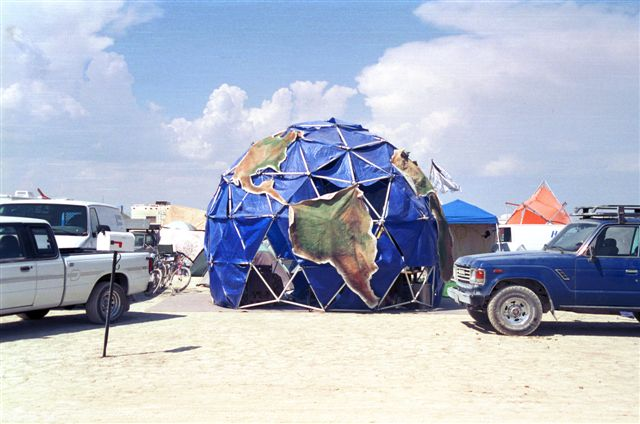## Geodesic domes

History and Terminology

A geodesic dome is a structure composed of a number of triangular facets, arranged in such a way as to approximate the surface of (a portion of) a sphere.

The term geodesic comes from the Greek geo-, earth, + daiesthai, to divide; thus we have "earth dividing" domes. A geodesic line connecting two points on a given surface is the shortest curve that connects them and is entirely contained on that surface. So if we use geodesic lines to connect the vertices of the triangles that form a geodesic sphere, we end up forming great circles that divide the sphere.

R. Buckminster Fuller is often credited with "inventing" the geodesic dome. In fact, although Fuller did popularize the geodesic dome, similar structures - also using subdivided icosohedra to approximate spheres - predated Fuller's 1954 patent by more than thirty years.

Some Basic Theory

To build a geodesic sphere, we start with an icosahedron - a regular polyhedron with twenty triangular faces. The vertices of the icosahedron are all equidistant from its center, so they determine a sphere. If we subdivide each of the triangular faces into smaller triangles, then some of the vertices of the smaller triangles lie inside the sphere rather than on it. By pushing these points radially outward from the icosahedron until they meet the sphere, we arrive at the vertices of our geodesic sphere.

The frequency of a geodesic dome is a measure of the number of triangles into which each face is subdivided. A 1-frequency dome (or sphere) is just (part of) an icosahedron; the faces are not subdivided at all. For a 2-frequency dome, the sides of each icosahedral face are divided in two, so that each face of the icoshedron is divided into 4 smaller triangles. For a 3-frequency dome, the sides are divided in three, so that the faces become 9 smaller triangles, and so on.

As a variation, we might start with one of the other regular polyhedra (there are only five) and choose some natural way of subdividing its faces into triangles.

Practice

Of course, the real reason you want to know anything at all about geodesic domes is so that you can build your own to take to Burning Man. After deciding on the materials you will use, the construction techniques, and the desired size of your dome, you will need to figure out the lengths of each of the struts that form your dome's triangular faces. As you can imagine from the brief discussion above, there will be quite a lot of trigonometry and arithmetic involved in making these calculations. Fortunately, if you are satisfied with a frequency of 6 or less, you can just use the marvelous Dome Calculator provided by the folks at Desert Domes.The dome featured in the image at the top of the page was constructed as a shade structure for Burning Man 2001. It's a 3-frequency dome with struts made out of inch-thick PVC pipe, and it has a radius of about 8 feet.

For Burning Man 2002, I built the dome up another level, to complete the top 15 faces of the icosahedron. Our neighbors camping nearby suggested that I should probably stop there, else our camp might roll away.

In keeping with Burning Man's "Floating World" theme for 2002, we also produced a globe-like covering for the dome, made out of vinyl and canvas tarp. So our dome became Continental Drift Camp.